# 机器学习实战（一）线性回归和局部加权线性回归

## 二、线性回归的数学推导

1.线性回归模型形如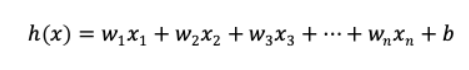• 注：线性回归得出的模型不一定是一条直线，在只有一个变量的时候，模型是平面中的一条直线；有两个变量的时候，模型是空间中的一个平面；有更多变量时，模型将是更高维的。
• 实际上，线性回归中通常使用残差平方和，即点到直线的平行于y轴的距离而不用垂线距离，残差平方和除以样本量n就是均方误差。均方误差作为线性回归模型的代价函数(cosfunction)。使所有点到直线的距离之和最小，就是使均方误差最小化，这个方法叫做最小二乘法。

2.代价函数：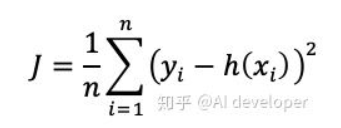3.偏导数法

• 偏导数法是非常麻烦的，需要一个一个地计算w。为了方便，这里以单变量线性回归为例。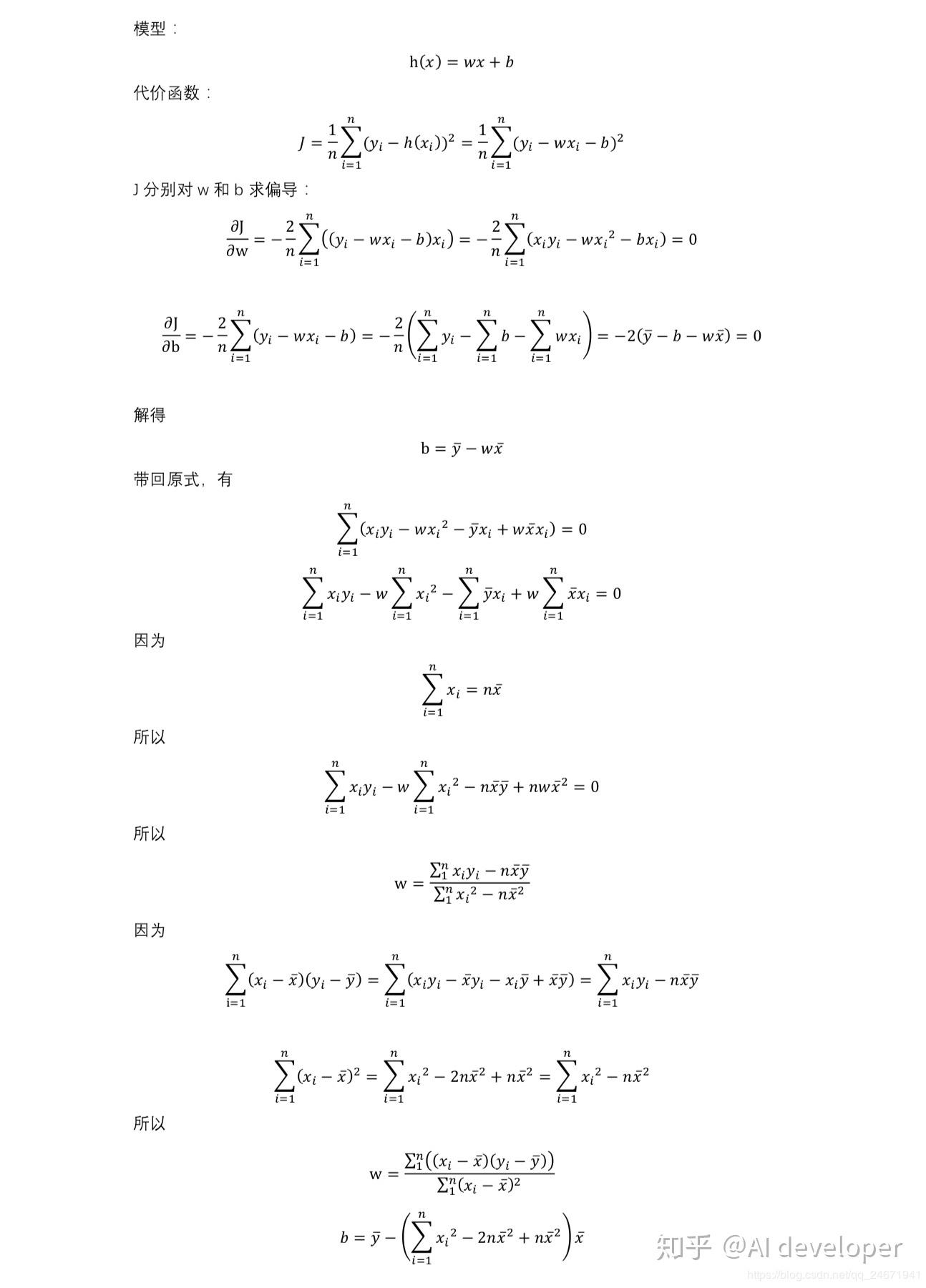4.正规方程法

• 正规方程使用矩阵运算，可以一次求出W向量。但是当变量(feature)个数大于数据个数时，会导致xTx不可逆，这时候就不能用此方法了。
• 使用正规方程法，如果希望得到的模型带有偏置项b，就要先给数据集X增加全为1的一列，这样才会把b包含在W中；如果不添加，那么模型是强制过原点的。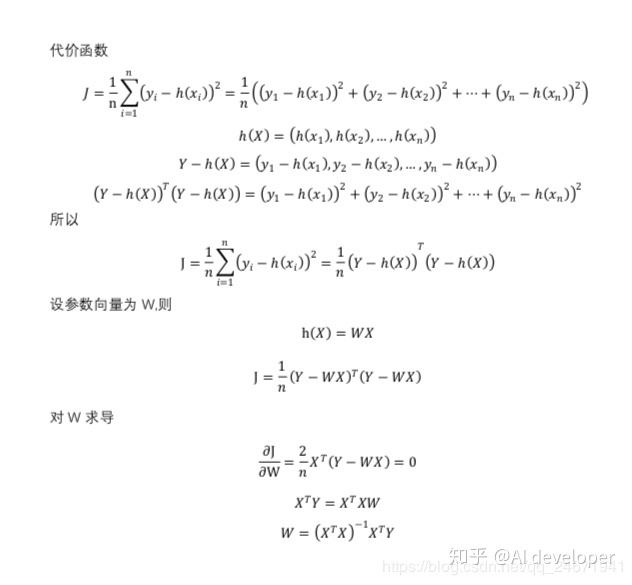## 三、标准线性回归

1.对于对给定数据集X和对应目标值y，线性回归目的是要找到回归系数w，使用线性方程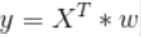来拟合这些数据点。
2.标准线性回归：

• 通常求取回归系数的做法是，求得使平方误差最下的w
• 平方误差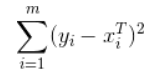• 解出回归系数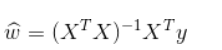#!/usr/bin/env python
# -*- coding:utf-8 -*-
# numpy函数库中的所有模块引入当前的命名空间
# 后者不建议使用，如果下次引用和numpy里的函数一样的情况，就会出现命名冲突。
from numpy import *
import matplotlib.pyplot as plt

# 该函数是用来打开一个用tab键分割的文本文件，默认文件每行的最后一个值是目标值

# 读出每行有n个数据，将n-1作为特征数,如果传入的txt文件是三列的，则numFeat = 2
dataMat = []
labelMat = []
# 打开文件
fr = open(fileName)
# 定义一个列表，来存取每一行的数据
lineArr = []
# 每行的数据根据tab键来划分，存到列表中
curLine = line.strip().split('\t')
# float()不能将整个list中的元素进行类型转换，所以用一个循环，一个元素一个元素地转换
for i in range(numFeat):
# 将n-1个特征组成一个新list，强制类型转换成float
lineArr.append(float(curLine[i]))
# 形成双层list形成一个矩阵
dataMat.append(lineArr)
# 将最后一个数值作为目标值
labelMat.append(float(curLine[-1]))
return dataMat, labelMat

# 计算最佳拟合直线
# 标准的线性回归函数，使用最小二乘法
def standRegres(yArr, xArr):
# 首先读入x和y并将它们保存到矩阵中
xMat = mat(xArr)
# 和transpose()一个意思
yMat = mat(yArr).T
# 计算x矩阵和x矩阵的转置乘积
xTx = xMat.T * xMat
# 使用的numpy中的linalg库，来计算行列式
# 计算系数向量w的最佳估计，其中要求xTx的逆，所以先要判断它是否为满秩矩阵（行列式不为0），
if linalg.det(xTx) == 0.0:
print("This matrix is singular, cannot do inverse222")
return
# 回归系数
ws = xTx.I * (xMat.T * yMat)
return ws, xMat, yMat

if __name__ == '__main__':
ws, xMat, yMat= standRegres(yArr, xArr)
yHat = xMat * ws

# 替换ax.scatter(xMat[:,1].flatten().A,yMat.T[:,0].flatten().A)
# 将xArr中第二列的数据添加进来，数组下标+1等于数组列数
for i in range(len(xArr)):
xcord.append(xArr[i])

# 对象，可以想象为一张画布；
fig = plt.figure()
# 将数据添加到画布上
ax.scatter(xcord,yArr)

xCopy = xMat.copy()
# 先将矩阵进行排序
xCopy.sort(0)
yHat = xCopy * ws

ax.plot(xCopy[: ,1], yHat, 'red')  # 画出拟合出的直线
plt.show()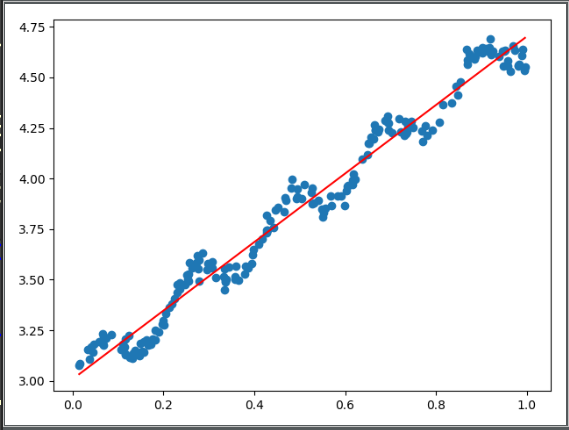## 四、局部加权线性回归（LWLR）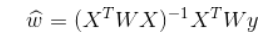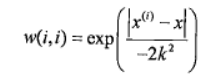#!/usr/bin/env python
# -*- coding:utf-8 -*-
from numpy import *
import matplotlib.pyplot as plt

# 局部加权线性回归，返回该条样本预测值
def lwlr(testPoint, xArr, yArr, k=1.0):
xMat = mat(xArr)
yMat = mat(yArr).T
m = shape(xMat)
# 创建为单位矩阵，再mat转换数据格式     因为后面是与原数据矩阵运算，所以这里是为了后面运算且不带来其他影响
weights = mat(eye((m)))
# 利用高斯公式创建权重W     遍历所有数据，给它们一个权重
for j in range(m):
# 高斯核公式1
diffMat = testPoint - xMat[j,:]
# 高斯核公式2    矩阵*矩阵.T 转行向量为一个值    权重值以指数级衰减
weights[j, j] = exp(diffMat * diffMat.T / (-2.0 * k ** 2))
# 求回归系数公式1
xTx = xMat.T * (weights * xMat)
# 判断是否有逆矩阵
if (linalg).det(xTx) == 0.0:
print("This matrix is singulat, cannot do inverse1111")
return
# 求回归系数公式2
ws = xTx.I * (xMat.T * (weights * yMat))
return testPoint * ws

# 循环所有点求出所有的预测值
def lwlrTest(testArr, xArr, yArr, k=1.0):
# 传入的k值决定了样本的权重，1和原来一样一条直线，0.01拟合程度不错，0.003纳入太多噪声点过拟合了
m = shape(testArr)
yHat = zeros(m)

# 返回该条样本的预测目标值
for i in range(m):
yHat[i] = lwlr(testArr[i], xArr, yArr, k)
return yHat
if __name__ == '__main__':
from linearRegression import regression
# 求所有预测值
yHat = lwlrTest(xArr, xArr, yArr, 0.01)
# 绘制数据点和拟合线（局部加权线性回归）
xMat = mat(xArr)
# 画拟合线 需要获得所有横坐标从小到大的坐标
srtInd = xMat[:,1].argsort(0)
# 获得排序后的数据
xSort = xMat[srtInd][:,0,:]

fig = plt.figure()
ax.plot(xSort[:,1],yHat[srtInd],color = 'red')
ax.scatter(xMat[:,1].flatten().A,mat(yArr).T.flatten().A)
plt.show()

yHat = lwlrTest(xArr, xArr, yArr, 0.003)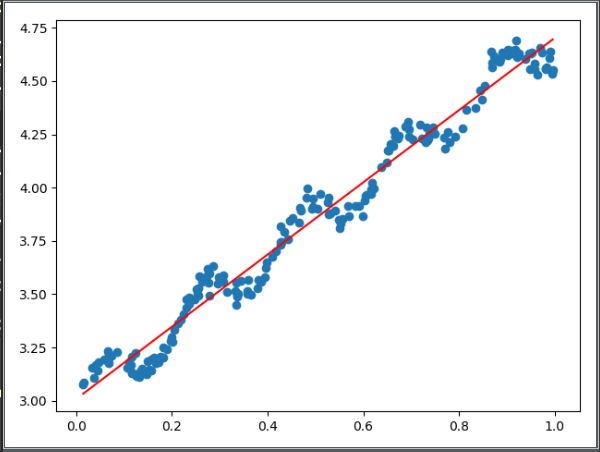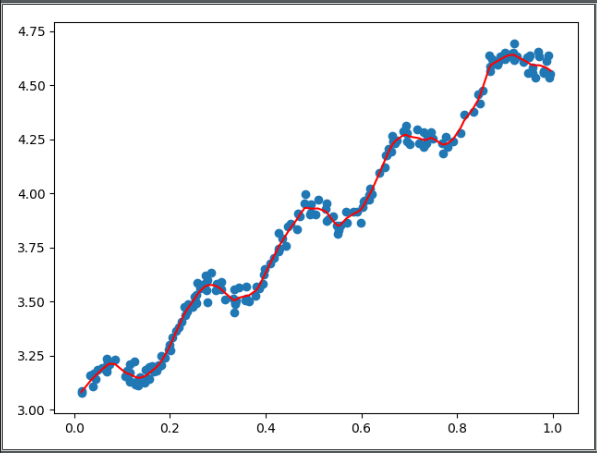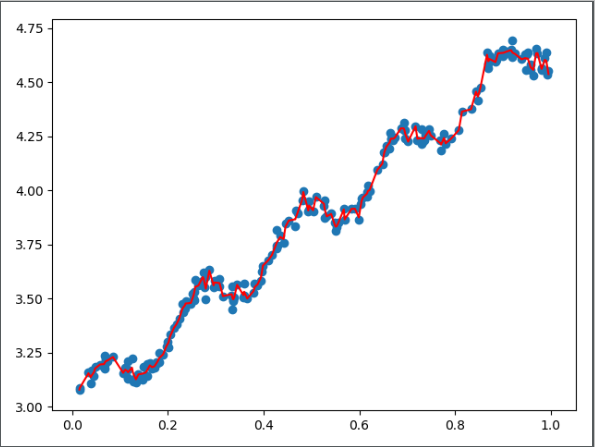## 五、总结

• step1：对于需要查询的输入x，找到其领域的训练样本
• step2：对于领域的训练集赋予更大的权重，求取回归系数w使得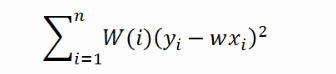• 最小，其中W(i)为权重值
• step3：预测输出：wx
• step4：对于新的输入，重复1-3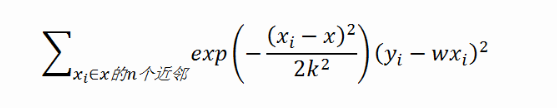## 六、示例：预测鲍鱼的年龄

# -*- coding:utf-8 -*-
import numpy as np

xArr = []
yArr = []
fr = open(fileName)
lineArr = []
curLine = line.strip().split('\t')
for i in range(numFeat):
lineArr.append(float(curLine[i]))
xArr.append(lineArr)
yArr.append(float(curLine[-1]))
return xArr, yArr

def lwlr(testPoint, xArr, yArr, k=1.0):
xMat = np.mat(xArr);
yMat = np.mat(yArr).T
m = np.shape(xMat)
# 创建权重对角矩阵
weights = np.mat(np.eye((m)))
# 遍历数据集计算每个样本的权重
for j in range(m):
diffMat = testPoint - xMat[j, :]
weights[j, j] = np.exp(diffMat * diffMat.T / (-2.0 * k ** 2))
xTx = xMat.T * (weights * xMat)
if np.linalg.det(xTx) == 0.0:
print("矩阵为奇异矩阵,不能求逆")
return
# 计算回归系数
ws = xTx.I * (xMat.T * (weights * yMat))
return testPoint * ws

def lwlrTest(testArr, xArr, yArr, k=1.0):
# 计算测试数据集大小
m = np.shape(testArr)
yHat = np.zeros(m)
# 对每个样本点进行预测
for i in range(m):
yHat[i] = lwlr(testArr[i], xArr, yArr, k)
return yHat

def standRegres(xArr, yArr):
xMat = np.mat(xArr);
yMat = np.mat(yArr).T
# 根据文中推导的公示计算回归系数
xTx = xMat.T * xMat
if np.linalg.det(xTx) == 0.0:
print("矩阵为奇异矩阵,不能求逆")
return
ws = xTx.I * (xMat.T * yMat)
return ws

return ((yArr - yHatArr) ** 2).sum()

if __name__ == '__main__':
print('训练集与测试集相同:局部加权线性回归,核k的大小对预测的影响:')
yHat01 = lwlrTest(abX[0:99], abX[0:99], abY[0:99], 0.1)
yHat1 = lwlrTest(abX[0:99], abX[0:99], abY[0:99], 1)
yHat10 = lwlrTest(abX[0:99], abX[0:99], abY[0:99], 10)

print('')

print('训练集与测试集不同:局部加权线性回归,核k的大小是越小越好吗？更换数据集,测试结果如下:')
yHat01 = lwlrTest(abX[100:199], abX[0:99], abY[0:99], 0.1)
yHat1 = lwlrTest(abX[100:199], abX[0:99], abY[0:99], 1)
yHat10 = lwlrTest(abX[100:199], abX[0:99], abY[0:99], 10)

print('')

print('训练集与测试集不同:简单的线性归回与k=1时的局部加权线性回归对比:')
ws = standRegres(abX[0:99], abY[0:99])
yHat = np.mat(abX[100:199]) * ws



《机器学习实战》

https://zhuanlan.zhihu.com/p/55094412?utm_source=qq&utm_medium=social&utm_oi=1091452863145926656

05-061万+
01-277705
10-181432
04-241万+
03-12100
04-091702
04-141242
01-168697
03-314483
04-02399
11-211420
04-22718
05-232666
08-221921
03-242526
10-24664
03-13603
05-15653
11-271911

### “相关推荐”对你有帮助么？

•非常没帮助
•没帮助
•一般
•有帮助
•非常有帮助被折叠的  条评论 为什么被折叠?到【灌水乐园】发言Qxw1012

¥2 ¥4 ¥6 ¥10 ¥20余额支付 (余额：-- )扫码支付获取中扫码支付点击重新获取扫码支付1.余额是钱包充值的虚拟货币，按照1:1的比例进行支付金额的抵扣。
2.余额无法直接购买下载，可以购买VIP、C币套餐、付费专栏及课程。余额充值